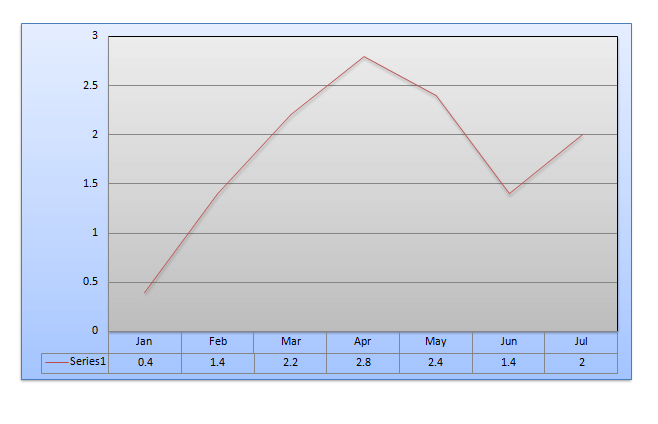Directions (Q. Nos. 1-4) Refer to the following line graph which represents the rainfall in- inches during the months of January through July in a particular year in a certain cityIn each of these questions, two quantities are given, one in Column A and one in Column B. Compare the two quantities.
(a) If the two quantities are equal
(b) If the quantity in column B is greater
(c) If the quantity in column A is greater
(d) If the relationship cannot be determined from the information given

Question 1.
Column A                                           Column B
The ratio of rainfall                                 2 : 3
in the months of
February and July

Question 2.
Column A                                            Column B
The average monthly                            1.9 inches
Rainfall recorded for the
7 months

Question 3.
Column A                                            Column B
25/2% of all the rain                          The amount of rainfall during the month of February
that fell during the 7
months period

Question 4.
Column A                                            Column B
The ratio between the                          The ratio between the rainfall in April and February
rainfall in May and January

 Month Rainfall January 0.4 February 1.4 March 2.2 April 2.8 May 2.4 June 1.4 July 2.0 Total 12.6

The ratio of rainfall in Feb : July = 1.4/2.0= 0.7:1 . The value of Column B is 2:3 = 0.67 : 1 . Since the value in column A is more than B, the correct option is (c)

 Month Rainfall January 0.4 February 1.4 March 2.2 April 2.8 May 2.4 June 1.4 July 2.0 Total 12.6

The average annual rainfall is 12.6/7 = 1.8 ins which is less than 1.9 inch. Since the value in column B is more Than A, the correct option is (b)

 Month Rainfall January 0.4 February 1.4 March 2.2 April 2.8 May 2.4 June 1.4 July 2.0 Total 12.6

Total rainfall in 7 months= 12.6= $12\frac{1}{2}%\,\,of\,\,12.6$ =$\frac{25\times 12.6}{200}=$ 1.575 inch

The amount of rainfall during the month of February = 1.4 inch

The value of the column A is more.

Therefore the correct option is (c).

Ratio of rainfall in May: January : 2.4:0.4 = 6:1

Ratio of rainfall in April : February : 2.8:1.4 = 2:1

The ratio of column A is more than the ratio of column B.

Therefore correct option is (c)